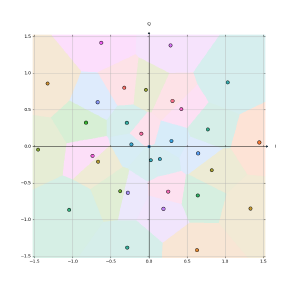matteo
/
constellationnet
Using autoencoders to optimize two-dimensional signal constellations for fiber optic communication systems
You can not select more than 25 topics Topics must start with a letter or number, can include dashes ('-') and can be up to 35 characters long.

#### 31 lines 679 B Raw Permalink Blame History

 `from constellation import util` `import torch` `from matplotlib import pyplot` `import matplotlib` `from mpl_toolkits.axisartist.axislines import SubplotZero` ``` ``` `# Number learned symbols` `order = 16` ``` ``` `# Color map used for decision regions and points` `color_map = matplotlib.cm.Dark2` ``` ``` `# File in which the trained model is saved` `input_file = 'output/constellation-order-{}.pth'.format(order)` ``` ``` `# Restore model from file` `model = torch.load(input_file)` `model.eval()` ``` ``` `# Setup plot` `fig = pyplot.figure()` `ax = SubplotZero(fig, 111)` `fig.add_subplot(ax)` ``` ``` `util.plot_constellation(` ` ax, model.get_constellation(),` ` model.channel, model.decoder,` ` grid_step=0.001, noise_samples=0` `)` ``` ``` ```pyplot.show() ``` ``` ```